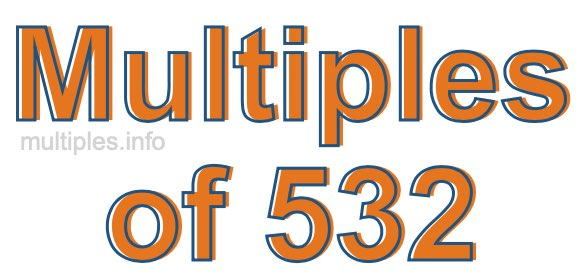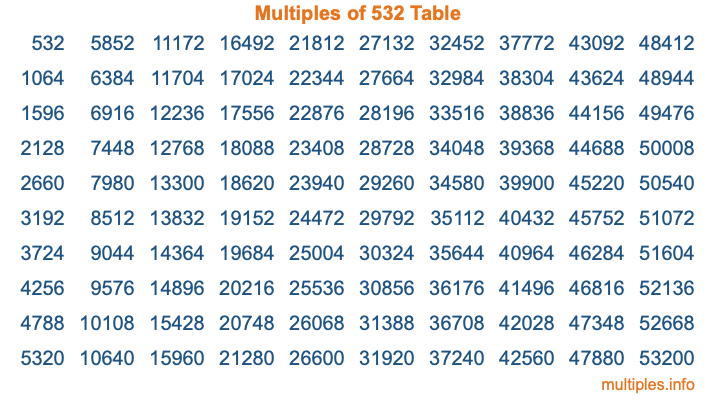Multiples of 532Welcome to the Multiples of 532 page. Here we will first teach you everything you will ever need to know about the multiples of 532, and then give you a study guide summary of everything we taught you to make sure you remember it all. Use this page to look up facts and learn information about the multiples of 532. This page will make you a multiples of five hundred thirty-two expert!

Definition of Multiples of 532
Multiples of 532 are all the numbers that when divided by 532 equal an integer. Each of the multiples of 532 are called a multiple. A multiple of 532 is created by multiplying 532 by an integer.

Therefore, to create a list of multiples of 532, you start with 1 multiplied by 532, then 2 multiplied by 532, then 3 multiplied by 532, and so on for as long as you want. Thus, the list of the first five multiples of 532 is 532, 1064, 1596, 2128, and 2660. To see a larger list of multiples of 532, see the printable image of Multiples of 532 further down on this page. We also have a category where you can choose any nth multiple of 532.

Multiples of 532 Checker
The Multiples of 532 Checker below checks to see if any number of your choice is a multiple of 532. In other words, it checks to see if there is any number (integer) that when multiplied by 532 will equal your number. To do that, we divide your number by 532. If the the quotient is an integer, then your number is a multiple of 532.

Is  a multiple of 532?

Least Common Multiple of 532 and ...
A Least Common Multiple (LCM) is the lowest multiple that two or more numbers have in common. This is also called the smallest common multiple or lowest common multiple and is useful to know when you are adding our subtracting fractions. Enter one or more numbers below (532 is already entered) to find the LCM.

Check out our LCM Calculator if you need more details about the Least Common Multiple or if you need the LCM for different numbers for adding and subtraction fractions.

nth Multiple of 532
As we stated above, 532 is the first multiple of 532, 1064 is the second multiple of 532, 1596 is the third multiple of 532, and so on. Enter a number below to find the nth multiple of 532.

th multiple of 532

Multiples of 532 vs Factors of 532
532 is a multiple of 532 and a factor of 532, but that is where the similarities end. All postive multiples of 532 are 532 or greater than 532. All positive factors of 532 are 532 or less than 532.

Below is the beginning list of multiples of 532 and the factors of 532 so you can compare:

Multiples of 532: 532, 1064, 1596, 2128, 2660, etc.

Factors of 532: 1, 2, 4, 7, 14, 19, 28, 38, 76, 133, 266, 532

As you can see, the multiples of 532 are all the numbers that you can divide by 532 to get a whole number. The factors of 532, on the other hand, are all the whole numbers that you can multiply by another whole number to get 532.

It's also interesting to note that if a number (x) is a factor of 532, then 532 will also be a multiple of that number (x).

Multiples of 532 vs Divisors of 532
The divisors of 532 are all the integers that 532 can be divided by evenly. Below is a list of the divisors of 532.

Divisors of 532: 1, 2, 4, 7, 14, 19, 28, 38, 76, 133, 266, 532

The interesting thing to note here is that if you take any multiple of 532 and divide it by a divisor of 532, you will see that the quotient is an integer.

Multiples of 532 Table
Below is an image of the first 100 multiples of 532 in a table. The table is in chronological order, column by column. The first column has the first ten multiples of 532, the second column has the next ten multiples of 532, and so on.The Multiples of 532 Table is also referred to as the 532 Times Table or Times Table of 532. You are welcome to print out our table for your studies.

Negative Multiples of 532
Although not often discussed or needed in math, it is worth mentioning that you can make a list of negative multiples of 532 by multiplying 532 by -1, then by -2, then by -3, and so on, to get the following list of negative multiples of 532:

-532, -1064, -1596, -2128, -2660, etc.

Multiples of 532 Summary
Below is a summary of important Multiples of 532 facts that we have discussed on this page. To retain the knowledge on this page, we recommend that you read through the summary and explain to yourself or a study partner why they hold true.

There are an infinite number of multiples of 532.

A multiple of 532 divided by 532 will equal a whole number.

532 divided by a factor of 532 equals a divisor of 532.

The nth multiple of 532 is n times 532.

The largest factor of 532 is equal to the first positive multiple of 532.

532 is a multiple of every factor of 532.

532 is a multiple of 532.

A multiple of 532 divided by a divisor of 532 equals an integer.

532 divided by a divisor of 532 equals a factor of 532.

Any integer times 532 will equal a multiple of 532.

Multiples of a Number
Here you can get the multiples of another number, all with the same attention to detail as we did for multiples of 532 on this page.

Multiples of
Multiples of 533
Did you find our page about multiples of five hundred thirty-two educational? Do you want more knowledge? Check out the multiples of the next number on our list!